(137 Kb)
Updated: 12-17-09 09:47 PM
Pictures
File Info
 Updated: 12-17-09 09:47 PM Created: 12-17-09 09:47 PM Downloads: 1,547 Favorites: 5 MD5:

# SciCalc

Version: 0.17
by: Balatist [More]

SciCalc is a full-featured scientific calculator with the ability to numerically solve equations, evaluate mathematical expressions, and plot functions.

Particularly, SciCalc can:

• Numerically solve equations.
• Store and recall user- and addon-defined variables.
• Calculate definite integrals and derivates.
• Determine p-values and z-values for normal distributions with arbitrary parameters.
• Determine the mean of a sequence of numbers.
• Interact with the game world to extract information such as amount of gold, honor, etc.
• Plot user-defined functions over a specified iterval and variable.

In addition, SciCalc has about 20 pre-programmed functions ranging from square-root and logarithms to trigonometric functions and factorials.

Type /calc or /scicalc for all functionality. Examples:

Evaluation:

/calc eval 1+log(10^2,10)+floor(pi)
Returns: SciCalc: 1+log(10^2,10)+floor(pi) = 6
/calc eval x = gcd(100,75)
Returns: SciCalc: x = gcd(100,75) = 25
/calc eval x^2
Returns: SciCalc: x^2 = 625

Calculus:

/calc integrate x^2+1,x,-2,2
Returns: SciCalc: Integral of x^2+1 over x=[-2,2] = 9.34
/calc diff x^3+1,x,1.23
Returns: SciCalc: Derivative of x^3+1 at x=1.23 = 4.54

Statistics:

/calc avg 1,2,3,4,5
Returns: SciCalc: Average = 3
/calc pval 0,1,3
Returns: SciCalc: P-value of 3 on N(0,1) = 0.001, Z-score = 3

Optional Files (0)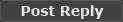Comment Options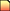12-18-09, 01:58 AM
Balatist
 Originally posted by Eidolarr I haven't played with it enough yet to be truly justified in commenting, but is there any chance you could pull most of the math out into a library for other addons to use? I don't think I've seen an advanced math library yet...
I'd be happy to do this. Just as an FYI until then, SciCalc should be a global and calling SciCalc:Evaluate(expr) where expr is simply a string will return a numeric result or nil on failure.

I'll look into moving the math functionality into a separate library.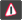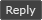12-18-09, 01:50 AM Eidolarr An Aku'mai Servant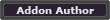Forum posts: 34 File comments: 32 Uploads: 3 I haven't played with it enough yet to be truly justified in commenting, but is there any chance you could pull most of the math out into a library for other addons to use? I don't think I've seen an advanced math library yet...12-17-09, 11:02 PM Polarina A Theradrim GuardianForum posts: 63 File comments: 12 Uploads: 2 Graphical representation. It would be nice if you could support graphical representation of the formulas.Report this File

Category Jump: